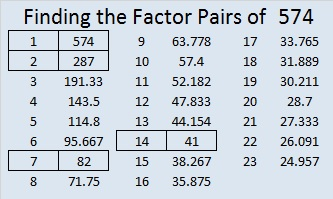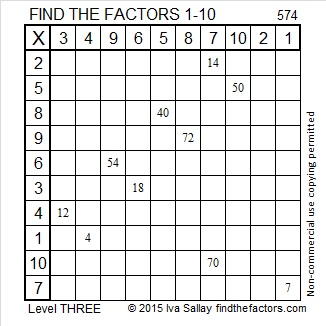# 574 Start at the top of the first column and fly down one cell at a time to solve this Level 3 puzzle

One of 574’s factors is the hypotenuse of a primitive Pythagorean triple so 574 is the hypotenuse of the triple 126-560-574. Which of 574’s factors is the greatest common factor of those three numbers?Print the puzzles or type the solution on this excel file: 10 Factors 2015-08-03

—————————————————————————————————

• 574 is a composite number.
• Prime factorization: 574 = 2 x 7 x 41
• The exponents in the prime factorization are 1, 1, and 1. Adding one to each and multiplying we get (1 + 1)(1 + 1)(1 + 1) = 2 x 2 x 2 = 8. Therefore 574 has exactly 8 factors.
• Factors of 574: 1, 2, 7, 14, 41, 82, 287, 574
• Factor pairs: 574 = 1 x 574, 2 x 287, 7 x 82, or 14 x 41
• 574 has no square factors that allow its square root to be simplified. √574 ≈ 23.958297.—————————————————————————————————

A Logical Approach to solve a FIND THE FACTORS puzzle: Find the column or row with two clues and find their common factor. Write the corresponding factors in the factor column (1st column) and factor row (top row).  Because this is a level three puzzle, you have now written a factor at the top of the factor column. Continue to work from the top of the factor column to the bottom, finding factors and filling in the factor column and the factor row one cell at a time as you go.